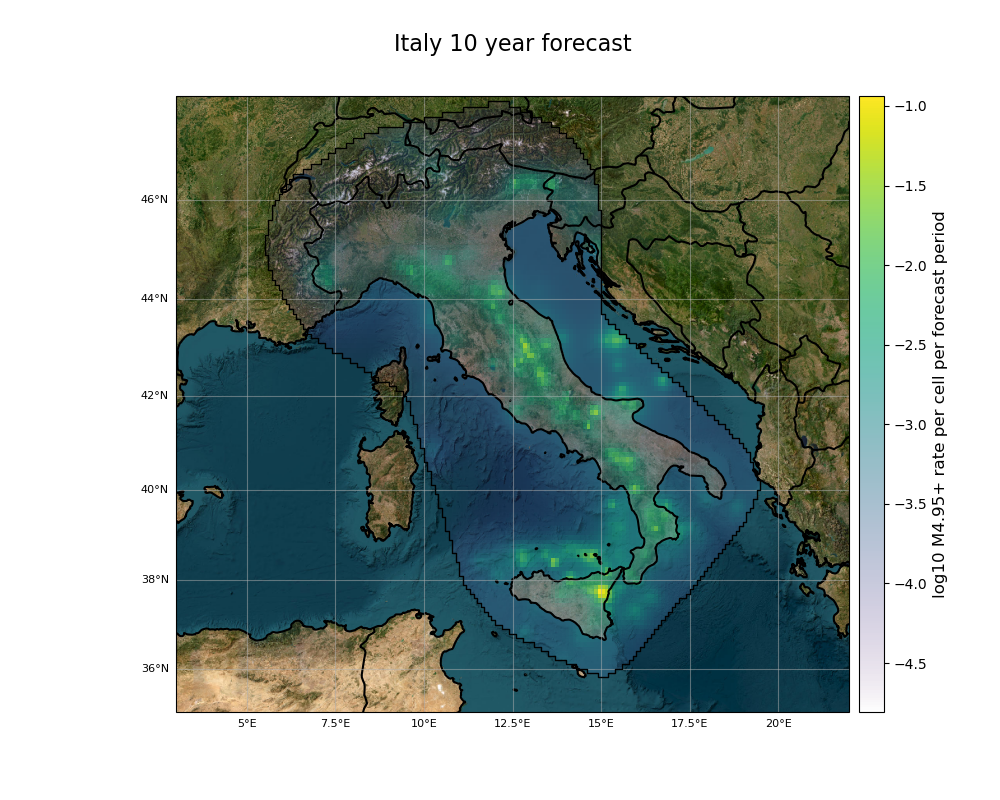Plot customizations

This example shows how to include some advanced options in the spatial visualization of Gridded Forecasts and Evaluation Results

Overview:
1. Define optional plotting arguments

2. Set extent of maps

3. Visualizing selected magnitude bins

4. Plot global maps

5. Plot multiple Evaluation Results

Example 1: Spatial dataset plot arguments

import csep
import cartopy
import numpy
from csep.utils import datasets, plots

import matplotlib.pyplot as plt

Load a Grid Forecast from the datasets

Selecting plotting arguments

Create a dictionary containing the plot arguments

args_dict = {'title': 'Italy 10 year forecast',
'grid_labels': True,
'borders': True,
'feature_lw': 0.5,
'basemap': 'ESRI_imagery',
'cmap': 'rainbow',
'alpha_exp': 0.8,
'projection': cartopy.crs.Mercator()}

These arguments are, in order:

• Assign a title

• Set labels to the geographic axes

• Draw country borders

• Set a linewidth of 0.5 to country borders

• Select ESRI Imagery as a basemap.

• Assign 'rainbow' as colormap. Possible values from from matplotlib.cm library

• Defines 0.8 for an exponential transparency function (default is 0 for constant alpha, whereas 1 for linear).

• An object cartopy.crs.Projection() is passed as Projection to the map

The complete description of plot arguments can be found in csep.utils.plots.plot_spatial_dataset()

Plotting the dataset

The map extent can be defined. Otherwise, the extent of the data would be used. The dictionary defined must be passed as argument

ax = forecast.plot(extent=[3, 22, 35, 48],
show=True,
plot_args=args_dict)Out:

/opt/hostedtoolcache/Python/3.7.12/x64/lib/python3.7/site-packages/cartopy/crs.py:228: ShapelyDeprecationWarning: __len__ for multi-part geometries is deprecated and will be removed in Shapely 2.0. Check the length of the geoms property instead to get the  number of parts of a multi-part geometry.
if len(multi_line_string) > 1:
/opt/hostedtoolcache/Python/3.7.12/x64/lib/python3.7/site-packages/cartopy/crs.py:280: ShapelyDeprecationWarning: Iteration over multi-part geometries is deprecated and will be removed in Shapely 2.0. Use the geoms property to access the constituent parts of a multi-part geometry.
for line in multi_line_string:
/opt/hostedtoolcache/Python/3.7.12/x64/lib/python3.7/site-packages/cartopy/crs.py:347: ShapelyDeprecationWarning: __len__ for multi-part geometries is deprecated and will be removed in Shapely 2.0. Check the length of the geoms property instead to get the  number of parts of a multi-part geometry.
if len(p_mline) > 0:

Example 2: Plot a global forecast and a selected magnitude bin range

Load a Global Forecast from the datasets

A downsampled version of the GEAR1 forecast can be found in datasets.

Filter by magnitudes

We get the rate of events of 5.95<=M_w<=7.5

We get the total rate between these magnitudes

rate_sum = rates_mw.sum(axis=1)

The data is stored in a 1D array, so it should be projected into region 2D cartesian grid.

Define plot arguments

We define the arguments and a global projection, centered at $lon=-180$

plot_args = {'figsize': (10,6), 'coastline':True, 'feature_color':'black',
'projection': cartopy.crs.Robinson(central_longitude=-179.0),
'title': forecast.name, 'grid_labels': False,
'cmap': 'magma',
'clabel': r'$\log_{10}\lambda\left(M_w \in [{%.2f},\,{%.2f}]\right)$ per '
r'${%.1f}^\circ\times {%.1f}^\circ$ per forecast period' %
(low_bound, upper_bound, forecast.region.dh, forecast.region.dh)}

Plotting the dataset To plot a global forecast, we must assign the option set_global=True, which is required by :ref:cartopy to handle internally the extent of the plotOut:

/opt/hostedtoolcache/Python/3.7.12/x64/lib/python3.7/site-packages/cartopy/crs.py:228: ShapelyDeprecationWarning: __len__ for multi-part geometries is deprecated and will be removed in Shapely 2.0. Check the length of the geoms property instead to get the  number of parts of a multi-part geometry.
if len(multi_line_string) > 1:
/opt/hostedtoolcache/Python/3.7.12/x64/lib/python3.7/site-packages/cartopy/crs.py:280: ShapelyDeprecationWarning: Iteration over multi-part geometries is deprecated and will be removed in Shapely 2.0. Use the geoms property to access the constituent parts of a multi-part geometry.
for line in multi_line_string:
/opt/hostedtoolcache/Python/3.7.12/x64/lib/python3.7/site-packages/cartopy/crs.py:347: ShapelyDeprecationWarning: __len__ for multi-part geometries is deprecated and will be removed in Shapely 2.0. Check the length of the geoms property instead to get the  number of parts of a multi-part geometry.
if len(p_mline) > 0:
/opt/hostedtoolcache/Python/3.7.12/x64/lib/python3.7/site-packages/cartopy/crs.py:239: ShapelyDeprecationWarning: Iteration over multi-part geometries is deprecated and will be removed in Shapely 2.0. Use the geoms property to access the constituent parts of a multi-part geometry.
line_strings = list(multi_line_string)
/opt/hostedtoolcache/Python/3.7.12/x64/lib/python3.7/site-packages/cartopy/crs.py:239: ShapelyDeprecationWarning: __len__ for multi-part geometries is deprecated and will be removed in Shapely 2.0. Check the length of the geoms property instead to get the  number of parts of a multi-part geometry.
line_strings = list(multi_line_string)
/opt/hostedtoolcache/Python/3.7.12/x64/lib/python3.7/site-packages/cartopy/crs.py:385: ShapelyDeprecationWarning: Iteration over multi-part geometries is deprecated and will be removed in Shapely 2.0. Use the geoms property to access the constituent parts of a multi-part geometry.
line_strings.extend(multi_line_string)
/opt/hostedtoolcache/Python/3.7.12/x64/lib/python3.7/site-packages/cartopy/crs.py:385: ShapelyDeprecationWarning: __len__ for multi-part geometries is deprecated and will be removed in Shapely 2.0. Check the length of the geoms property instead to get the  number of parts of a multi-part geometry.
line_strings.extend(multi_line_string)

Example 3: Plot a catalog

start_time = csep.utils.time_utils.strptime_to_utc_datetime('1995-01-01 00:00:00.0')
end_time = csep.utils.time_utils.strptime_to_utc_datetime('2015-01-01 00:00:00.0')
min_mag = 3.95
catalog = csep.query_comcat(start_time, end_time, min_magnitude=min_mag, verbose=False)

# **Define plotting arguments**
plot_args = {'basemap': 'ESRI_terrain',
'markersize': 2,
'markercolor': 'red',
'alpha': 0.3,
'mag_scale': 7,
'legend': True,
'legend_loc': 3,
'mag_ticks': [4.0, 5.0, 6.0, 7.0]}

Out:

These arguments are, in order:

• Assign as basemap the ESRI_terrain webservice

• Set minimum markersize of 2 with red color

• Set a 0.3 transparency

• mag_scale is used to exponentially scale the size with respect to magnitude. Recommended 1-8

• Set legend True and location in 3 (lower-left corner)

• Set a list of Magnitude ticks to display in the legend

The complete description of plot arguments can be found in csep.utils.plots.plot_catalog()

# **Plot the catalog**
ax = catalog.plot(show=False, plot_args=plot_args)Example 4: Plot multiple evaluation results

Load L-test results from example .json files (See Grid-based Forecast Evaluation for information on calculating and storing evaluation results)

L_results = [csep.load_evaluation_result(i) for i in datasets.l_test_examples]
args = {'figsize': (6,5),
'title': r'$\mathcal{L}-\mathrm{test}$',
'title_fontsize': 18,
'xlabel': 'Log-likelihood',
'xticks_fontsize': 9,
'ylabel_fontsize': 9,
'linewidth': 0.8,
'capsize': 3,
'hbars':True,
'tight_layout': True}

Description of plot arguments can be found in plot_poisson_consistency_test(). We set one_sided_lower=True as usual for an L-test, where the model is rejected if the observed is located within the lower tail of the simulated distribution.

ax = plots.plot_poisson_consistency_test(L_results, one_sided_lower=True, plot_args=args)

# Needed to show plots if running as script
plt.show()Total running time of the script: ( 1 minutes 16.252 seconds)

Gallery generated by Sphinx-Gallery